Video Tutorial Enthalpy of Solution

Quick Notes Enthalpy of Solution

• When an ionic compound dissolves in water, an enthalpy change occurs and is called enthalpy of solution.
• Energy is required to break apart the solid lattice into free ions and energy is released when these ions are 'hydrated' with water molecules.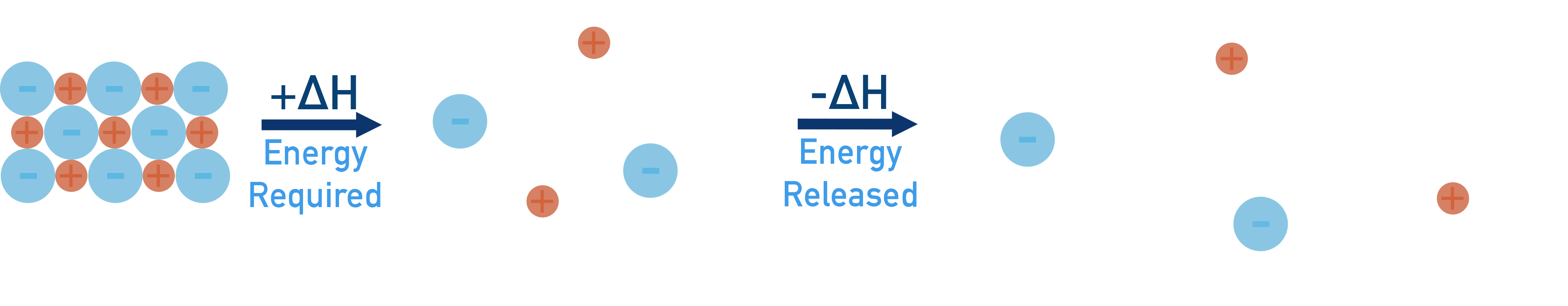• If the energy required to break apart the lattice is greater than the energy released by hydration, enthalpy of solution is endothermic (+ΔH).
• If the energy required to break apart the lattice is smaller than the energy released by hydration, enthalpy of solution is exothermic (-ΔH).
• Enthalpy of solution is the enthalpy change that occurs when 1 moles worth of an ionic lattice dissolves completely in water (to form an infinitely dilute solution).
• Enthalpy of hydration is the enthalpy change that occurs when 1 moles worth of gaseous ions dissolve completely in water (to form an infinitely dilute solution).
• Enthalpy of hydration is always an exothermic process (-ΔH).
• Lattice enthalpy of dissociation is the enthalpy change that occurs when 1 moles worth of an ionic compound is converted into its constituent, gaseous ions.
• Lattice enthalpy of dissociation is always an endothermic process (+ΔH).
• Lattice dissociation enthalpy is the opposite of lattice enthalpy of formation - lattice enthalpy of formation has same value, but is exothermic (-ΔH).
• Can use born-harber cycles with enthalpies of solution to find lattice enthalpies indirectly.

Full Notes Enthalpy of Solution

When an ionic lattice dissolves in water, an energy change will occur and the water temperature will change.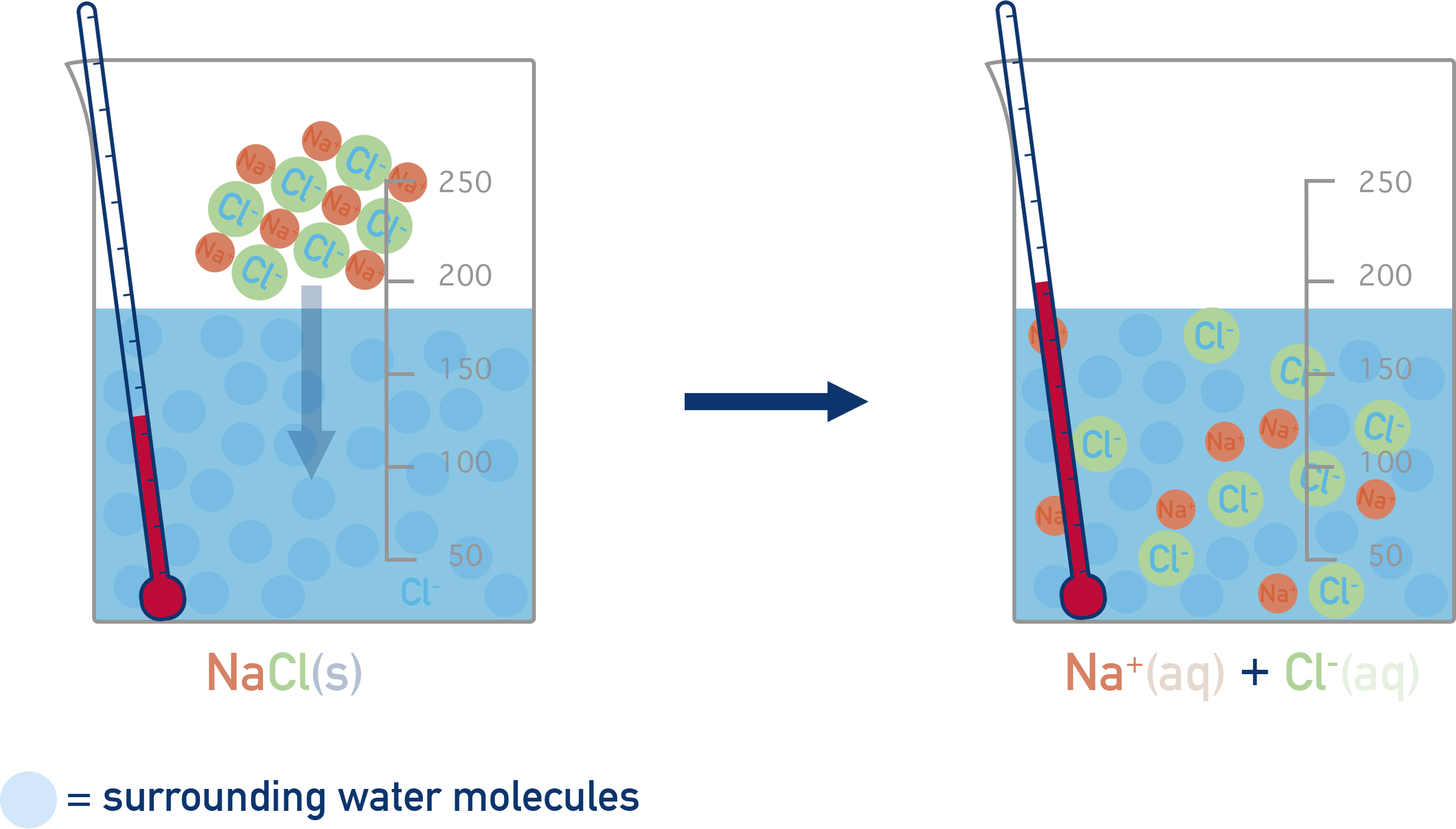For some compounds, the process is exothermic (water temperature increases) and for others, it is endothermic (water temperature decreases). We can explain these energy changes by considering the dissolving as a two stage process.

Step 1 - breaking the attraction forces holding the lattice together to free the ions in the solid

Step 2 - forming new forces of attraction between free ions and water molecules

To break apart the solid structure (step 1) and overcome the attraction forces between ions, energy is required (positive enthalpy change, +ΔH).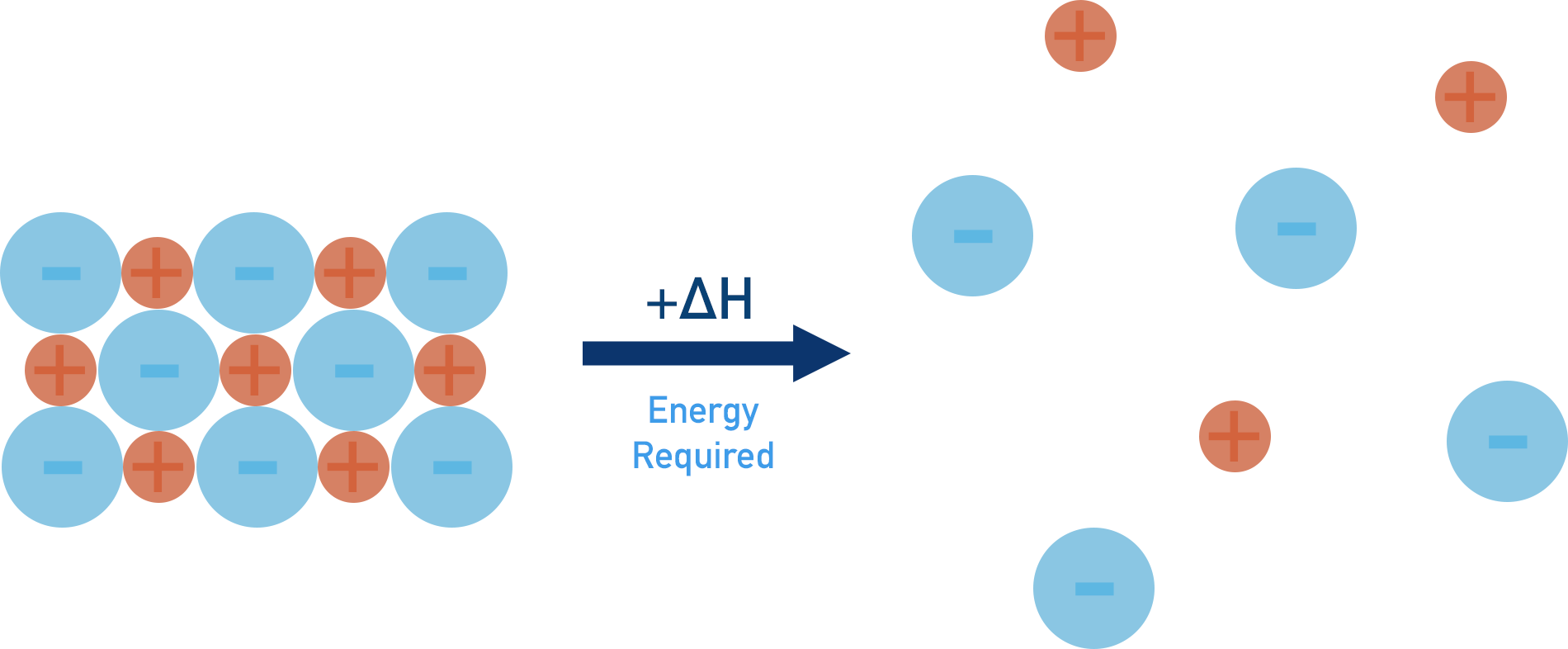The forming of new forces between ions and water molecules (step 2) releases energy (negative enthalpy change, -ΔH). This is referred to as ‘hydration’ and the ions end up hydrated. We show this as the state (aq).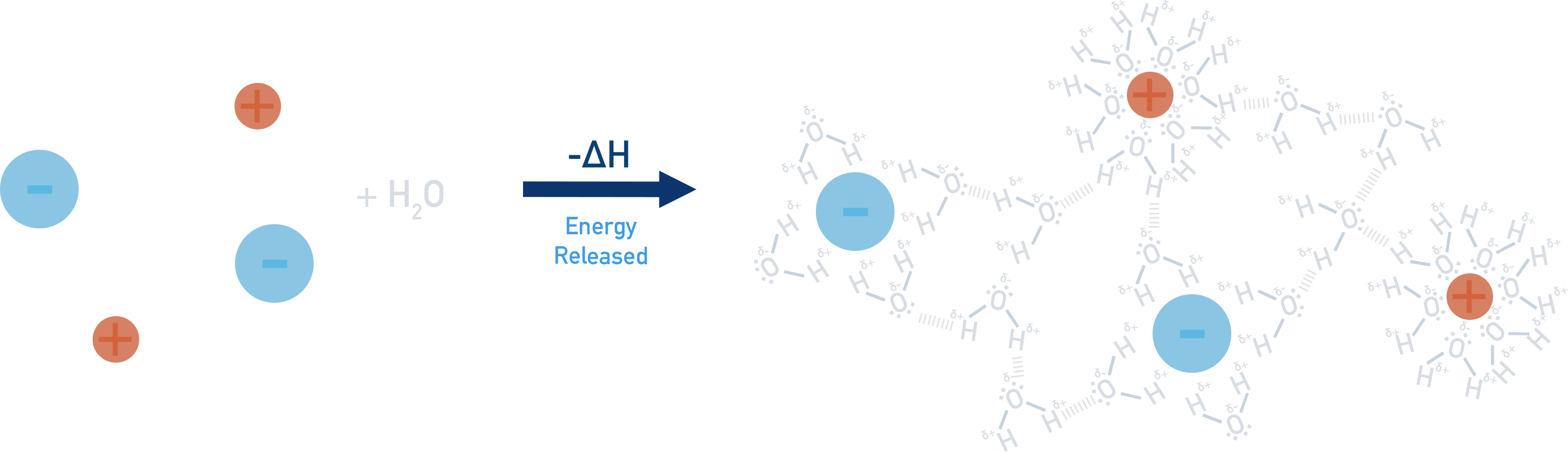When a solid actually dissolves, the above two steps pretty much happen at the same time and it is not possible to separate them into two stages. Forces of attraction between ions are overcome as new forces of attraction between ions and water molecules are formed.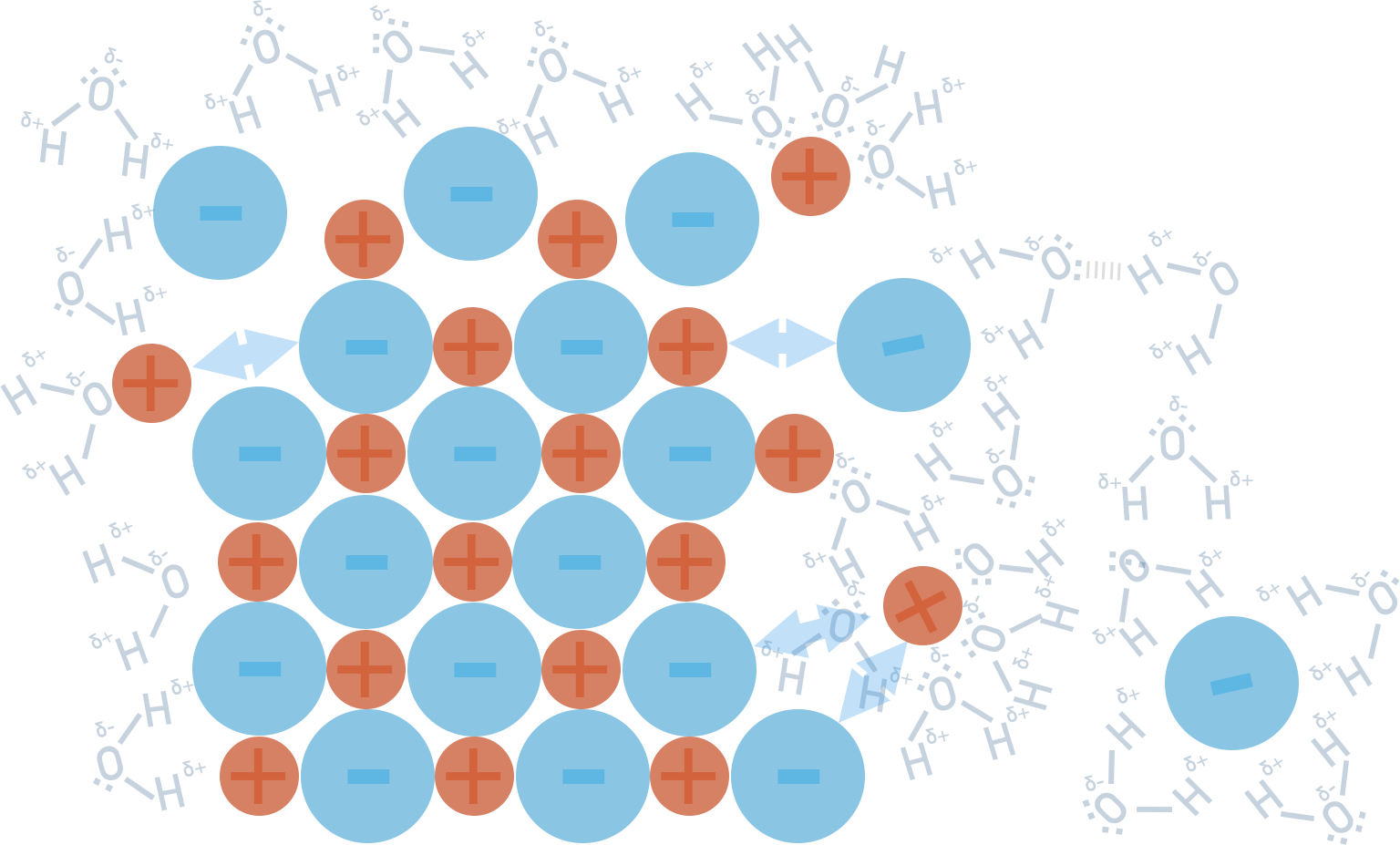As a result, we simply measure the overall enthalpy change that occurs and this is called ‘enthalpy of solution’. Remember however, that this enthalpy change is as a result of breaking the ionic bonding in the lattice and forming new forces between water molecules.

Standard enthalpy of solution

The enthalpy change that occurs when 1 mole of solute is dissolved completely in water, to form an infinitely dilute solution. Measured at standard conditions.

Note – infinitely dilute means that the addition of any more water molecules would have no impact on the enthalpy change that is occurring.

## Using Enthalpies of Solution

We can use enthalpies of solution to find lattice enthalpies of formation*.

Using Hess’ Law (see Hess’ Cycles), we can take two routes for the hydration of ions in an ionic compound and construct a Born-Haber cycle. Both routes start with an ionic lattice (s) and end with hydrated (aq) ions.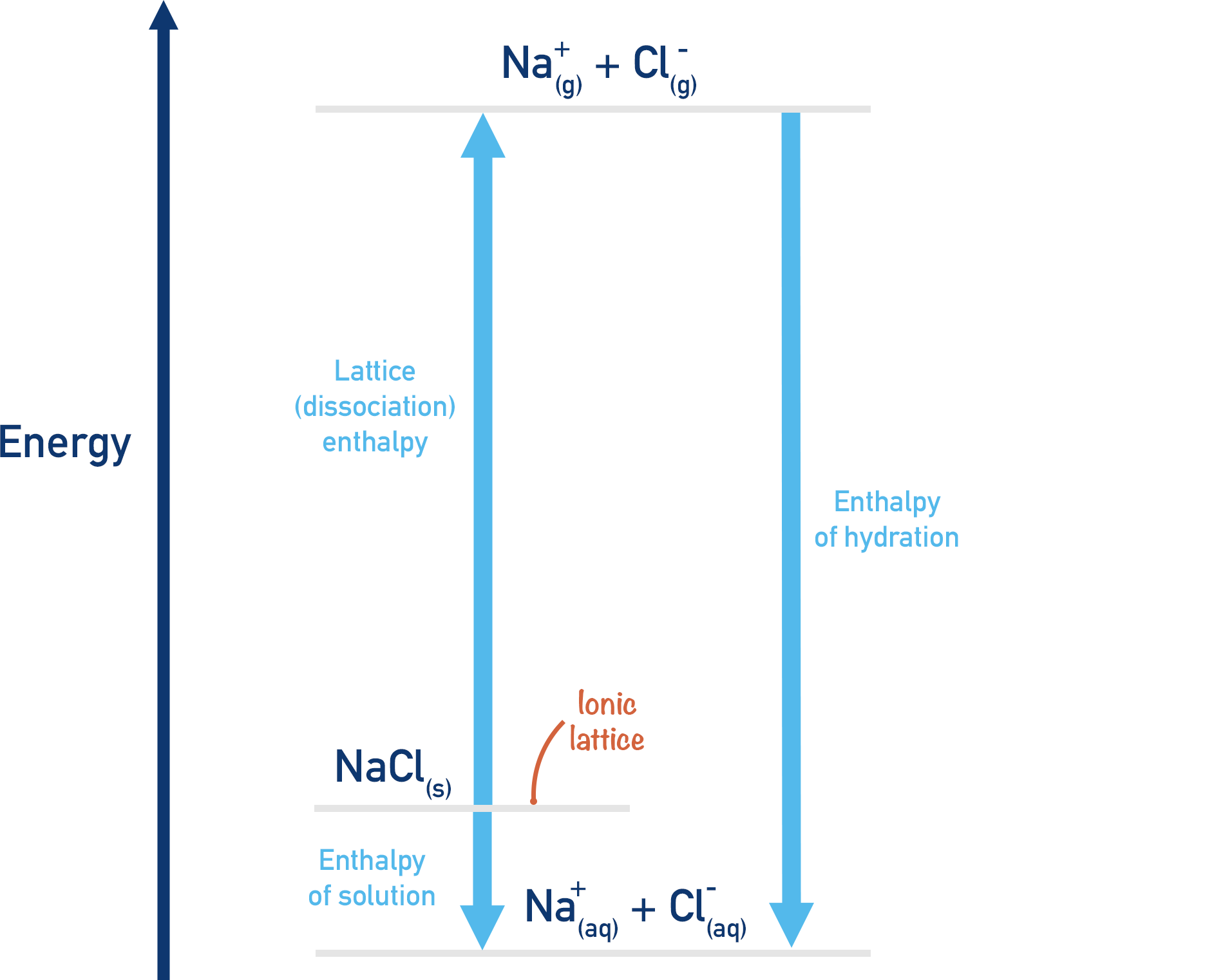Route 1:

Dissolve the ionic compound in water (enthalpy of solution). We'll use sodium chloride (NaCl) as an example.

Enthalpy of solution

NaCl(s) → Na+(aq) + Cl-(aq)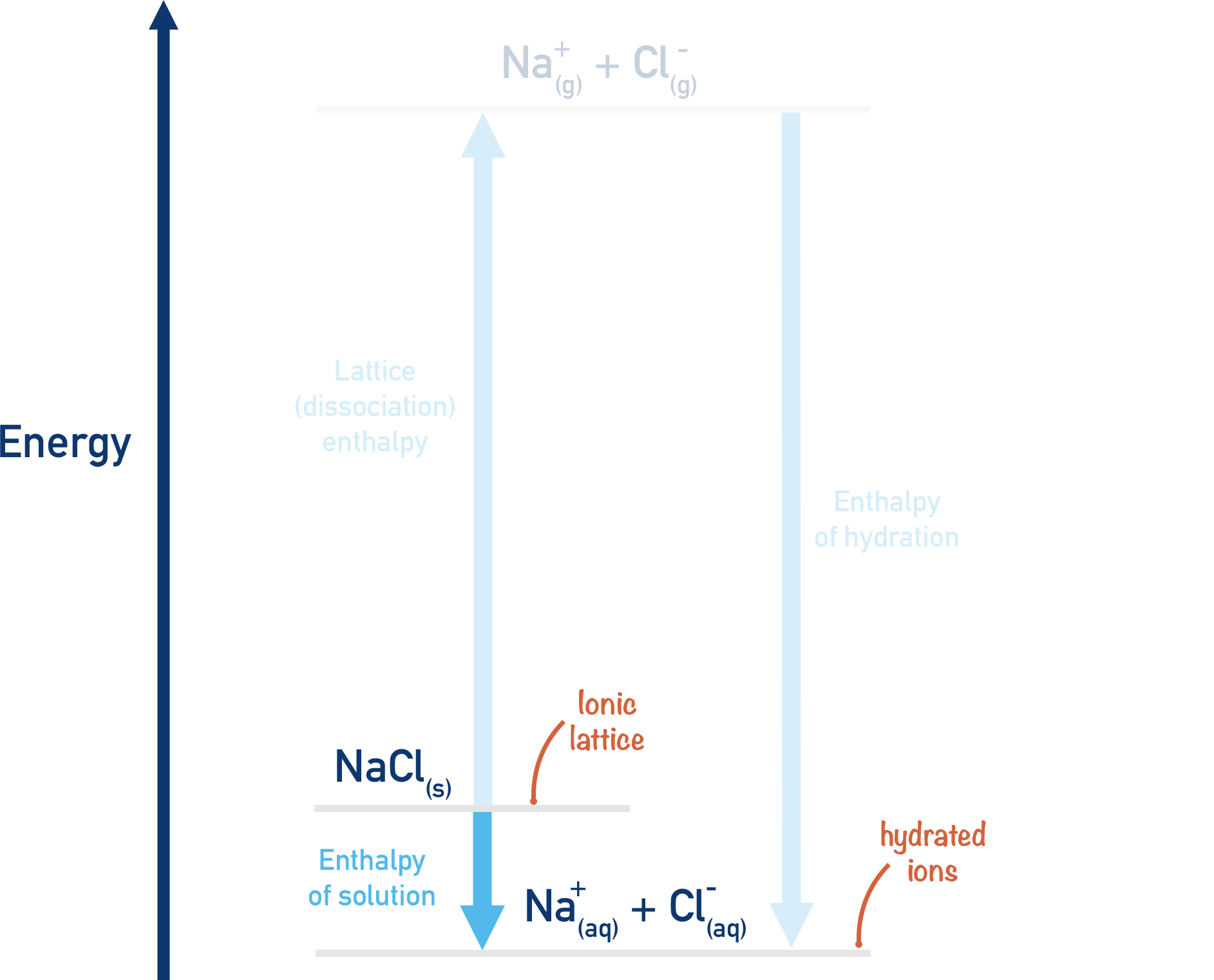Route 2:

Break apart ionic lattice (lattice dissociation - ), and release free, gaseous ions. These gaseous ions can then be mixed with water to become hydrated and the enthalpy change that occurs measured – ‘enthalpy of hydration’.

Lattice enthalpy of dissociation

NaCl(s) → Na+(g) + Cl-(g)

Enthalpy of hydration

Na+(g) + Cl-(g) → Na+(aq) + Cl-(aq)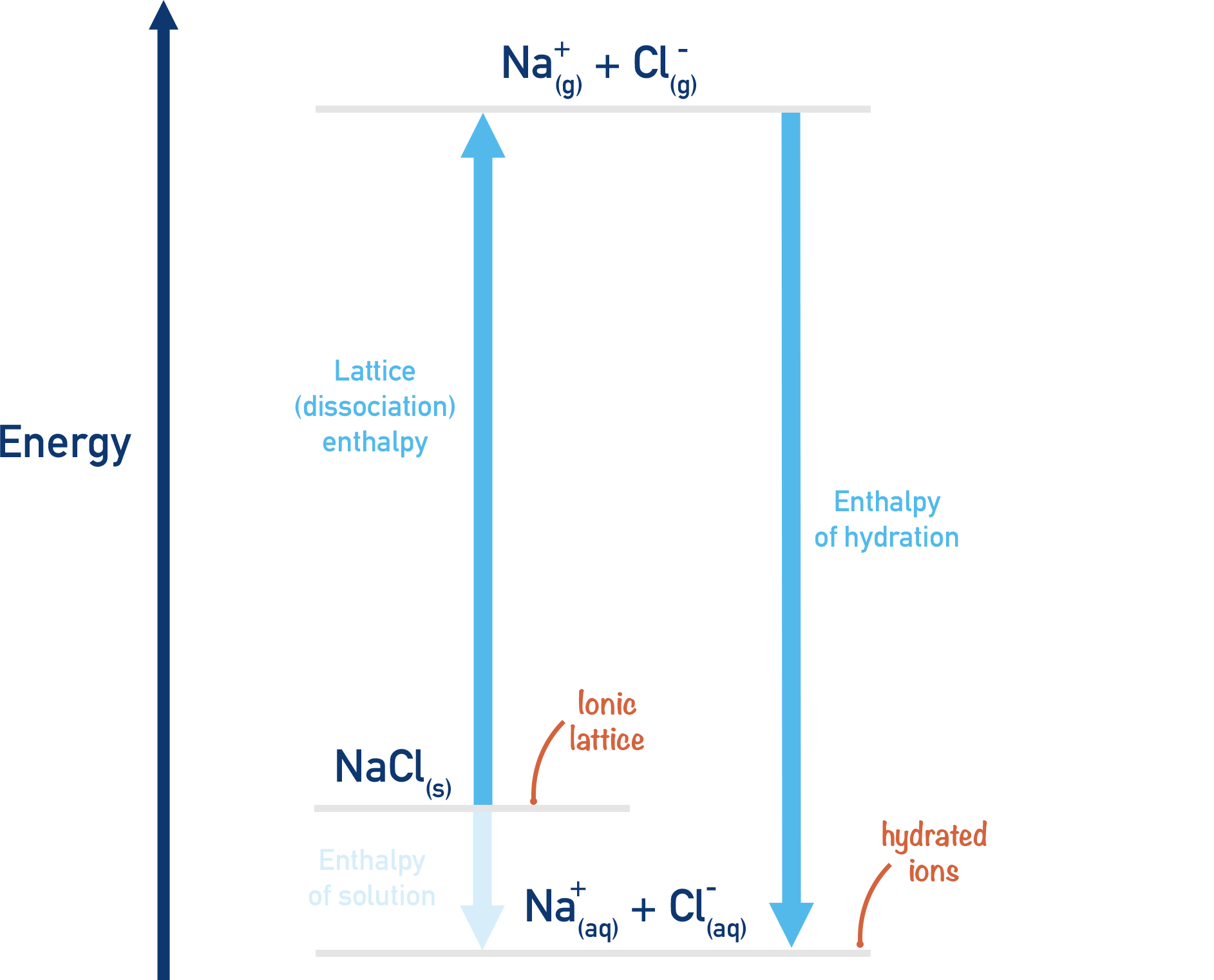The start, NaCl(s) and end, Na+(aq) and Cl-(aq) of both route 1 and 2 are the same. Using Hess's Law we can see that:Meaning...Meaning...Meaning...*see below

If more energy is required to break apart the lattice than is released when the ions are hydrated, the enthalpy of solution will be endothermic.

If less energy is required to break apart the lattice than is released when the ions are hydrated, the enthalpy of solution will be exothermic.

*Lattice enthalpies are defined as the enthalpy change that occurs when one moles worth of ionic lattice is formed from its constituent gaseous ions. When using enthalpies of solution, we are really referring to the lattice dissociation enthalpy – this is the enthalpy change that occurs when one moles worth of an ionic lattice is converted into its constituent, gaseous ions.

Lattice dissociation enthalpies and lattice enthalpies for a particular compound have the same value, they just have opposite enthalpy changes. Lattice enthalpies are always exothermic (negative enthalpy change, -ΔH) and Lattice dissociation enthalpies are always endothermic (positive enthalpy change, +ΔH).